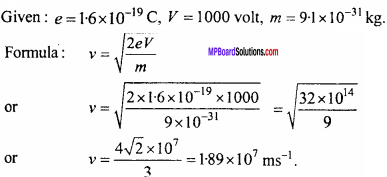# MP Board Class 12th Physics Important Questions Chapter 11 Dual Nature of Radiation and Matter

## MP Board Class 12th Physics Important Questions Chapter 11 Dual Nature of Radiation and Matter

### Dual Nature of Radiation and Matter Important Questions

Dual Nature of Radiation and Matter Objective Type Questions

Question 1.
Choose the correct answer of the following:

Question 1.
The charge of electron is:
(a) 1.6 x 10-19C
(b) 1.6 x 10-9C
(c) Zero
(d) 9.1 x 10-31C.
(b) 1.6 x 10-9C

Question 2.
The energy acquired by an electron which is accelerated by a potential difference of 10 volt is:
(a) 10 eV
(b) 10 joule
(c) 1 eV
(d) 1 joule.
(a) 10 eV

Question 3.
Electron was discovered by:
(a) Planck
(b) Milikan
(c) J. J. Thomson
(d) Ruther Ford.
(c) J. J. ThomsonQuestion 4.
The momentum of photon of energy E is:
(a) $$\frac {E}{c}$$
(b) Ec
(c) $$\frac {e}{E}$$
(d) $$\sqrt { Ec }$$
(a) $$\frac {E}{c}$$

Question 5.
The de Broglie wavelength associated with an electron accelerated by 150 volt is:
(a) 150 Å
(b) I mU
(c) 1 nm
(d) 1 Å
(d) 1 Å

Question 2.
Match the columns:
I.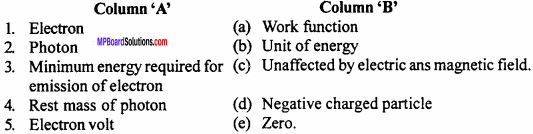1. (d)
2. (c)
3. (a)
4. (e)
5. (b)

II.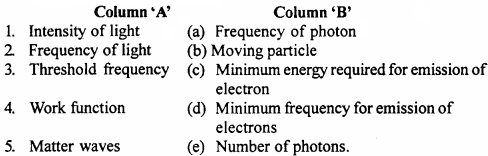1. (e)
2. (c)
3. (d)
4. (c)
5. (b)

III.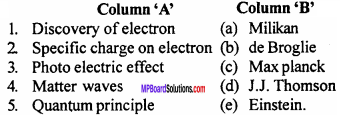1. (d)
2. (a)
3. (e)
4. (b)
5. (c)

IV.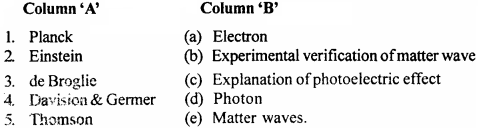1. (d)
2. (c)
3. (e)
4. (b)
5. (a)

Question 3.
Fill in the blanks:

1. The phenomenon of emission of electron from metallic surface is called ……………..
2. The minimum amount of energy required for emission of electron from a metallic surface is called ……………..
3. The phenomenon of emission of electrons from the metallic surface on heating it is called ……………..
4. The electrons are emitted from metallic surface when a light of specific frequency is incident on it. This phenomenon is called ………………
5. The maximum value of photo current in Lenard’s experiment is called ……………..
6. In Lenard’s experiment the negative voltage corresponding to which the photoelectric current becomes zero is called ……………..
7. ……………..is that minimum frequency below which the electron can not be emitted from metal surface what so ever be the intensity.
8. The minimum amount of energy which is required for emission of electrons from a metal is called ……………..
9. The radiation has particle and wave nature both. This nature is called as ……………..
10. A wave is associated with energy moving particle it is called as ……………..
11. 1 eV = …………….. joule.
12. The rest mass of an electron is ……………..
13. The minimum frequency which is required for emission of electrons from a metal surface is called ……………..
14. The matter waves are discovered by ……………..
15. The kinetic energy of photoelectrons depends upon the …………….. of incident light.

1. Electron emission
2. Work function
3. Thermionic emission
4. Photoelectric effect
5. Saturated current
6. Cut off potential
7. Threshold frequency
8. Work function
9. Dual nature
10. Debroglie or matter waves
11. 1.6 x 10-19J
12. 9.1 x 10-31kg
13. Threshold frequency
14. De Broglie
15. Frequency.

Question 4.
Write the question in one word/sentence:

1. Who discovered the photoelectric effect?
2. Who discovered the electron?
3. State the name of phenomena which shows the particle nature of radiations.
4. On what factors does the maximum velocity of emitted electrons depend?
5. Who explain first the photoelectric effect?
6. Write value of 1 eV in joule.
7. Write formula of de – Broglie wavelength.
8. What is rest mass of photons?

1. Hertz
2. J.J. Thomson
3. Photoelectric effect and Compton effect
4. The frequency of incident radiation and work function of metal
5. Albert Einstein
6. 1 eV = 1.602 x 10-19J
7. λ = $$\frac {h}{mv}$$
8. Zero

Question 1.
What is mass of a photon of frequency u?
From the formula:
E = mc2 = hu
m = $$\frac { hu }{ { c }^{ 2 } }$$

Question 2.
Can the photon exist in the state of rest?
No.

Question 3.
Which metals are usually used for photoelectric emission?
Alkali metals because their work function is low.

Question 4.
The energy of a photon is hu and its momentum is $$\frac {h}{λ}$$ , what will be the of photon?
The velocity of photon v = $$\frac {E}{P}$$ = $$\frac {hu}{h/λ}$$
or v = uλ.Question 5.
On what factors does the work function of a metal depend?
The work function of a substance depends on its nature.

Question 6.
Due to which radiations the photoelectric effect is produced in metal?

Question 7.
How many joule are in one electron volt?
1 eV = 1.6 x 1019J.

Question 1.
What is photon nature of light? Explain.
The light consists of small bundles of energy. Each bundle is called ‘quanta’ or ‘photon’. Each photon has a definite energy. This energy is given by the equation E = hv, where h is Planck’s constant and u is frequency.

Question 2.
What are de – Broglie waves? Write de – Broglie equation.
The waves which are associated with matter are called matter waves or de – Broglie waves
Equation λ = $$\frac {h}{mv}$$
Where λ wavelength, h → Planck’s constant
m → mass, u → velocity of wave.

Question 3.
Why the wave nature of matter is not observed in daily life?
de Broglie wavelength is given by
λ = $$\frac {h}{mv}$$
∴ λ ∝ $$\frac {1}{m}$$
As the mass is large, the wavelength is smaller. Therefore, the effect cannot be observed in daily life.Question 4.
The threshold wavelength of sodium metal is 6800 Å. Clarify the statement
The sodium metal will emit the electrons when a light of wavelength 6800 Å will fall on it. If the wavelength of light is more than this value, it will not emit the electrons.

Question 5.
Write properties of matter waves.

1. The matter waves are not electromagnetic in nature. Electromagnetic waves are produced only by charged particles.
2. The wavelength of matter waves is independent of the charge or nature of particle.
3. For lighter particle, the wavelength of matter waves will be greater.

Question 6.
What is dual nature of radiations?
In same experiments light behaves as particles while in same other experiments it behaves as wave. In the other words either the light radiations will behave as particle or wave but not simultaneously. This is called dual nature of light.

Question 7.
The Planck’s constant is h and frequency of a photon is v then write the Einstein’s photoelectric equation.
If h is Planck’s constant and ν is frequency of photon, then Einstein’s photo – electric equation is
h(ν – ν0) = $$\frac {1}{2}$$mvmax2

Question 8.
What is specific charge of electron?
The specific charge of electron is 1.76 x 1011C/kg.

Question 9.
What is cut off potential?
The negative potential of anode for which the photoelectric current becomes zero is called stopping potential or cut off potential.

Question 10.
What do you mean by specific charge of a charged particle? What is its value for electron?
The ratio of charge on a particle and its mass is called as specific charge of that particle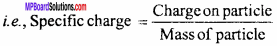For electron specific charge is 1.76 x 1011C/kg.

Question 11.
Explain meaning of Planck’s constant, write its formula. Also write its value.
Planck’s constant:
According to quantum theory the energy of a photon of a radiation is proportional to its frequency i.e.,
E ∝ ν
or E = hv
Where h is a proportionality constant called as Planck’s constant
Thus, h = $$\frac {E}{ν}$$
Hence, the ratio of energy of photon to the frequency of photon is called Plancks’ constant.
The value of h is 6.6 x 10-34J/sec.

Question 12.
What do you mean by electron – volt? Find its value in joule.
The energy gained by an electron, when it is accelerated by 1 volt potential difference is called one electron – volt.
Let the charge on an electron is e and it is accelerated by V volt.
∴ Work done = eV
Energy of electron = eV
or 1 eV = 1.6 x 10-19 x 1
= 1.6 x 10-19 joule.

Question 13.
What is work function? The work function of Lithium and Copper are 2.3 e V and 4 eV respectively, which will be useful for visible light?
The least energy which can eject the electrons from the surface of the metal, is called its work function. The work function of lithium is less, therefore it is useful for the visible light.Question 14.
What is thermionic emission?
The phenomenon of emission of electrons, from the surface of the metal when it is heated, is called thermionic emission.

Question 15.
What properties should possess by a metal used for thermionic emission?
The metal used for thermionic emission should have the following properties:

1. Its work function should be small, so that even at low temperature the electrons can be emitted.
2. The melting point should be high.

Question 16.
What is the reason that the photoelectric emission do not take place for a wavelength, greater than a definite value from a metal?
For the emission of electrons from a metal a minimum energy is required which is called work function.
Work function ϕ = $$\frac { hc }{ { \lambda }_{ 0 } }$$
Where, λ0 is threshold wavelength.
If the wavelength of incident light is greater than λ0 then the energy of radiation will be less than work function and the emission of electron will not be possible.

Question 17.
What do you mean by threshold frequency? What is the relation between work function and threshold frequency?
The threshold frequency is that minimum frequency of light, below which the photoelectrons do not emit, what so ever may be the intensity of light.
The relation between work function and threshold frequency is
ϕ = hν0
Where, h is Planck constant.

Question 18.
What is photoelectric effect? How it was explained by Einstein?
Photoelectric effect:
When some photo sensitive substances are exposed to ultraviolet light electrons are ejected from their surface which are called photoelectrons. This process is called photoelectric emission. On the basis of equation Emax = hν – ϕ0 Einstein gave explanations of laws of photoelectric effect.

1. If the intensity of incident light of constant frequency is increased then the number of electrons emitted per second from the metallic surface will increase but since energy of each photon is hv then the energy of emitted electron will remain constant (or same).

2. By the Einstein’s equation it is clear that E ∝ u, hence on increasing the frequency of incident photons, the energy of emitted electrons will increase.

3. If the frequency of incident photons is less than threshold frequency then electrons will not emit from the metal surface what so even be the intensity.

Question 19.
In the photoelectric effect the occurrence of threshold frequency give more importance to photon theory as compared to the wave theory. Explain.
According to wave theory for the light of all frequencies the photoelectric effect should be possible but according to Einstein’s photoelectric equation the kinetic energy of emitted electron is $$\frac {1}{2}$$mv2 = h(ν – ν0). If the frequency o of the incident photons is less than threshold frequency u0. Then the K.E. of electrons will be negative. But it is not possible.

Hence it is clear that the emission of electrons is not possible if the frequency is less than threshold energy. Thus it is clear that the occurrence of threshold frequency in photoelectric effect give more importance to photon theory’ as compared to the wave theory.

Question 20.
The energy of each electrons ejected from the metal surface is different even if the incident light of same frequency is exposed on it. Why?
The electrons which are on the surface of metal experience smaller attraction force due to positive ions. While the electrons which are ejected from inner part of the metal experience greater force of attraction. Therefore the velocity of surface electrons are greater hence their energies are greater.

Question 21.
Show that for an electron which is accelerated by a potential difference V, the value of de – Broglie wavelength is given by X = $$\frac { 12.27 }{ \sqrt { V } }$$ Å
Let the kinetic energy of a moving particle of mass m is K.
Then, K = $$\frac {1}{2}$$mv2
or 2mK = m2v2
or mv = $$\sqrt { 2mK }$$
From the formula λ = $$\frac {h}{mv}$$
or λ = $$\frac { h }{ \sqrt { 2mK } }$$ … (1)
If a charge q is accelerated by a potential difference V the kinetic energy of thw charged particle be
K = qV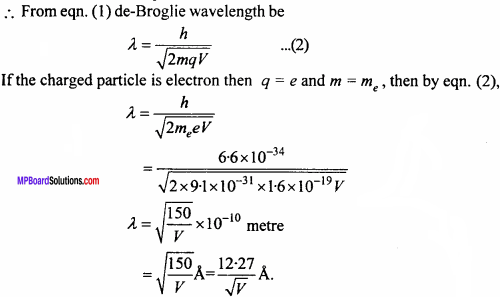Question 22.
Explain meaning of work function in photoelectric effect by giving the equation.
In the photoelectric effect if maximum kinetic energy of emitted electron is $$\frac {1}{2}$$mv2maxenergy of incident photon is hν and work function is ϕ then the relation between them can be given by
$$\frac {1}{2}$$mv2max hνϕ
If hν = ϕ, then $$\frac {1}{2}$$mv2max = 0. Thus it is clear that if the energy of incident light is equal to work function then the velocity of emitted electron will be zero.
Again if ϕ > hν, then $$\frac {1}{2}$$mv2max will be negative. which is not possible.
Thus, the work function is that minimum energy which is essential for the emission of electrons.

Question 23.
Write photoelectric effect and explain that what are the reasons due to which wave theory could not explain the photoelectric effect?
Photoelectric effect:
When some photo sensitive substances are exposed to ultraviolet light electrons are ejected from their surface which are called photoelectrons. This process is called photoelectric emission. On the basis of equation Emax = hν – ϕ0 Einstein gave explanations of laws of photoelectric effect.

1. If the intensity of incident light of constant frequency is increased then the number of electrons emitted per second from the metallic surface will increase but since energy of each photon is hv then the energy of emitted electron will remain constant (or same).

2. By the Einstein’s equation it is clear that E ∝ u, hence on increasing the frequency of incident photons, the energy of emitted electrons will increase.

3. If the frequency of incident photons is less than threshold frequency then electrons will not emit from the metal surface what so even be the intensity.

The photoelectric effect could not be explained by the wave theory because :

1. By wave theory, energy of light is distributed uniformly and measured in terms of intensity of beam. By increasing the intensity, the amplitude and energy of waves will increases. If light beam falls on the metal, the energy accumulated in these waves should be transferred uniformly to the electrons on the surface and so they must be emitted out.

Waves having higher intensity will impart more energy to the electrons and so the energy of the photoelectrons must increase. But this is against the experimental observation that the maximum K.E. of the photoelectrons does not depend on the intensity of light.

2. The energy transferred by the light waves will not go to a particular electron, but it will be distributed among all the electrons. Hence, electrons will take some time in accumulating energy required for emission. But practically, the emission of electrons takes place immediately after the light is incident on the metal.

Question 24.
Is the photoelectric effect an instant action? The K.E. of electron and photon are same which will have longer wavelength?
Yes, photoelectric effect is an instant action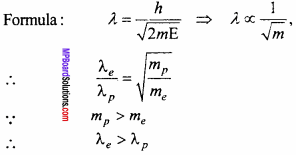Thus the electron will have longer de – Broglie wavelength.

Question 25.
Define de – Broglie matter wave and obtain expression λ = $$\frac { h }{ \sqrt { 2mE} }$$ for de – Broglie wavelength.
de – Broglie waves:
The waves which are associated with matter are called matter waves or de – Broglie waves.
If a particle of mass m is moving with velocity v then its kinetic energy be
E = $$\frac {1}{2}$$mv2
Multiplying by 2m on both sides
2mE = m2v2
or 2mE = (mv)2
or 2mE = p2, (∵ p=mv)
∴ The de – Broglie wavelength associated with the matter particle be
λ = $$\frac {h}{p}$$
or λ = $$\frac { h }{ \sqrt { 2mE}}$$

Question 26.
The propagation of light is by the photons even then it is not seen discontinuous why?
The effect of a photon on the retina remains for $$\frac {1}{16}$$ seconds but when the light enters into eye, about 1018 photons strike the retina per second. Therefore it does not appear discontinuous.

Question 27.
What is the mass of a photon of frequency ν and wavelength λ? What is rest mass of a photon?
The mass of photon of frequency ν
E = mc2 = hν
Then m = $$\frac { hν }{ { c }^{ 2 } }$$
The mass of photon of wevelength λ
E = mc2 = $$\frac {hc}{λ}$$
or m $$\frac {h}{cλ}$$
The rest mass of photon is zero.Question 28.
What is photoelectric effect? Derive Einstein’s formula:
$$\frac {1}{2}$$mv2max = hν – hν0
Or
Establish Einstein’s equation relating to the photoelectric emission.
Photoelectric effect:
When some photo sensitive substances are exposed to ultraviolet light electrons are ejected from their surface which are called photoelectrons. This process is called photoelectric emission. On the basis of equation Emax = hν – ϕ0 Einstein gave explanations of laws of photoelectric effect.
1. If the intensity of incident light of constant frequency is increased then the number of electrons emitted per second from the metallic surface will increase but since energy of each photon is hv then the energy of emitted electron will remain constant (or same).

2. By the Einstein’s equation it is clear that E ∝ u, hence on increasing the frequency of incident photons, the energy of emitted electrons will increase.

3. If the frequency of incident photons is less than threshold frequency then electrons will not emit from the metal surface what so even be the intensity.

Derivation:
Einstein explained photoelectric effect by Planck’s constant quantum theory. According to which light rays travel from one place to the other in the form of bundles of energy. These are called photons. Energy of each photon is hu and momentum is $$\frac {h}{λ}$$.
Where, h → Planck constant, u → Frequency of light and λ → Wavelength of light. When photon of energy hu incident on any substance, its energy is used in two ways :

1. Part of energy is used to eject the electron from the surface, which is called work function and represented by ϕ and is different for different substances.

2. Remaining energy is used to increase the kinetic energy of emitted electron.

Therefore, hv = ϕ + Ek … (1)
Where, EK → Maximum kinetic energy of electron.
If u0 is a threshold frequency and maximum velocity of electron is vmax.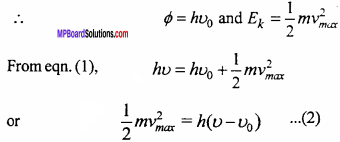Eqn. (2) is called Einstein’s equation.

Question 29.
Explain the following:

1. Thermionic emission
2. Threshold frequency
3. Work function
4. Matter waves.

1. Thermionic emission : The phenomenon of emission of electrons from the surface of metal when it is heated is called thermionic emission.

2. Threshold frequency : The threshold frequency is that minimum frequency of light, below which the photoelectrons do not emit what so even be the intensity of light.

3. Work function : The minimum energy which can eject the electrons from the surface of the metal is called work function.

4. Matter waves: The waves which are associated with matter are called matter waves or de – Broglie waves.

Question 1.
What is photoelectric effect? Write the laws of photoelectric emission.
Or
State any two laws of photoelectric effect?
Or
What is photoelectric effect?
Photoelectric effect:
When some photo sensitive substances are exposed to ultraviolet light electrons are ejected from their surface which are called photoelectrons. This process is called photoelectric emission. On the basis of equation Emax = hν – ϕ0 Einstein gave explanations of laws of photoelectric effect.

1. If the intensity of incident light of constant frequency is increased then the number of electrons emitted per second from the metallic surface will increase but since energy of each photon is hv then the energy of emitted electron will remain constant (or same).

2. By the Einstein’s equation it is clear that E cc u, hence on increasing the frequency of incident photons, the energy of emitted electrons will increase.

3. If the frequency of incident photons is less than threshold frequency then electrons will not emit from the metal surface what so even be the intensity.

Laws of photoelectric emission:

1. There is a definite cut off value of frequency below which electrons cannot be ejected by any substance.
2. Number of emitted electrons are directly proportional to the intensity of light incident.
3. Kinetic energy of emitted electrons depends on the frequency of incident light on substance.
4. There is no time logging between the incident of light and emission of electrons.Question 2.
Write about an electron, proton and neutron under following points:

1. Chemical symbol
2. Charge
3. Name of discoverer.

1. Symbols:

• Electron – -1
• Proton – 1H1
• Neutron – on1

2. Charge:

• Electron – – 1.6 x 10-19coulomb
• Proton – +1.6 x 10 coulomb
• Neutron – Zero.

3. Name of the discoverer:

• Electron – J.J. Thomson (1897)
• Proton – Goldstein (1896)
• Neutron – James Chadwik (1932).

Question 3.
Determine de – Broglie relation.
Or
What are de – Broglie waves? Write down de – Broglie wave equation.
de – Broglie wave : The waves which are associated with matter are called matter waves or de – Broglie waves.

Wave equation : According to quantum theory, the energy of a photon is given by the formula:
E = hν … (1)
Where, h = Planck constant and u= Frequency of light. Suppose the mass of photon is m.
By Einstein’s mass energy relation,
E = mc2 … (2)
Where, c = Velocity of light.
Now, from eqns. (1) and (2), we get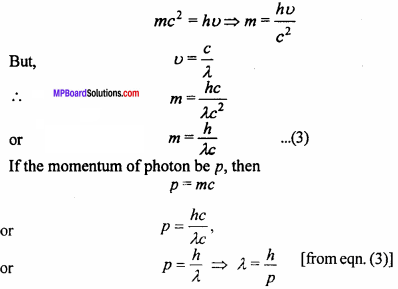Which is called de – Broglie wave equation.
Again, if the velocity of mass m is v, then
p = mv and λ = $$\frac {h}{mv}$$
Which is de – Broglie relation.

Question 4.
Prove that the de – Broglie wavelength λ of a matter particle of energy E is given λ = $$\frac { h }{ \sqrt { 2mE } }$$
The waves which are associated with matter are called matter waves or de – Broglie waves.
If a particle of mass m is moving with velocity v then its kinetic energy be
E = $$\frac {1}{2}$$mv2
Multiplying by 2m on both sides
2mE = m2v2
or 2mE = (mv)2
or 2mE = p2, (∵ p=mv)
∴ The de – Broglie wavelength associated with the matter particle be
λ = $$\frac {h}{p}$$
or λ = $$\frac { h }{ \sqrt { 2mE}}$$Question 5.
What are photons? Write any four properties of photons.
An electro magnetic wave travels in the form of discrete packets on bundles of energy called quanta. One quanta of light radiation is called photon.

Properties:

1. The speed of photons in vacuum is equal to speed of light.
2. Photons are electrically neutral. They are not affected by the electric or magnetic fields.
3. The energy of photons is expressed in terms of electron volt (eV).
4. The energy of photons is given by E = hν = $$\frac {hc}{ λ}$$
5. Where, h is Planck’s constant, ν is frequency and λ is wavelength.

Dual Nature of Radiation and Matter Numerical Questions

Question 1.
An electron is accelerated upto 100 volt. Find its kinetic energy.
Solution:
Kinetic energy = eV,
∴ e = 1.6 x 10-19 and V= 100 volt
∴ Kinetic energy = 1.6 x 10-19 x 100 joule
= 100 eV

Question 2.
Find energy of photon of wavelength 4000 A.
Solution:
Given: λ = 4000Å = 4000 x 10-10
or λ = 4 x 10-7
Formula: E = hν or E = $$\frac {hc}{λ}$$
Where h (Planck’s constant) = 6.6 x 10-34 J sec c
= 3 x 108 m/sec
Putting the values in the formula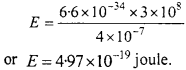Question 3.
An electron is accelerated by a potential difference of 1000 volt, what will be its velocity?
Solution: